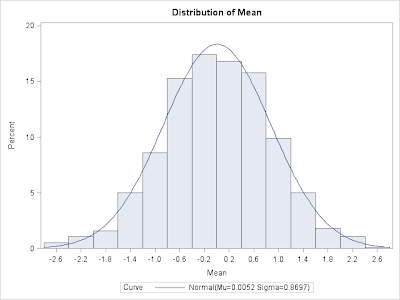## Monday, October 31, 2011

### Example 9.12: simpler ways to carry out permutation testsIn a previous entry, as well as section 2.4.3 of the book, we describe how to carry out a 2 group permutation test in SAS as well as with the coin package in R. We demonstrate with comparing the ages of the female and male subjects in the HELP study.

In this entry, we revisit the permutation test using other functions.

R

We describe a simpler interface to carry out and visualize permutation tests using the functions from the mosaic package.

We begin by replicating our previous example (section 2.6.4, p. 87).
`ds = read.csv("http://www.math.smith.edu/r/data/help.csv")library(coin)numsim = 1000oneway_test(age ~ as.factor(female),   distribution=approximate(B=numsim-1), data=ds)`

which yields the following output:
` Approximative 2-Sample Permutation Testdata:  age by as.factor(female) (0, 1) Z = -0.9194, p-value = 0.3894alternative hypothesis: true mu is not equal to 0 `

We conclude that there is minimal evidence to contradict the null hypothesis that the two groups have the same ages back in their respective populations.

Now we demonstrate another way to run this test in a more general form, using the mosaic package's do() function combined with the * operator to repeatedly carry out fitting a linear model with a parameter for female which will calculate our test statistic (difference in means between females and males) repeatedly after shuffling the group indicators. The shuffle() function permutes the group labels, and then the summary statistic is calculated.
`> library(mosaic)> obsdiff = with(ds, mean(age[female==1]) - mean(age[female==0]))> obsdiff     mean 0.7841284 > summary(age ~ female, data=ds, fun=mean)age    N=453+-------+---+---+--------+|       |   |N  |mean    |+-------+---+---+--------+|female |No |346|35.46821||       |Yes|107|36.25234|+-------+---+---+--------+|Overall|   |453|35.65342|+-------+---+---+--------+`

Now we can run the permutation test, then display the results on a souped-up histogram with different shading for values larger in magnitude than the observed statistic (see above).
`res = do(numsim) * lm(age ~ shuffle(female), data=ds)pvalue = sum(abs(res\$female) > abs(obsdiff)) / numsimxhistogram(~ female, groups = abs(female) > abs(obsdiff),   n=50, density=TRUE, data=res, xlab="difference between groups",  main=paste("Permutation test result: p=", round(pvalue, 3)))`

The results are similar to those from the previous test: there is little evidence to contradict the null hypothesis.

SAS

In SAS, we'll take another approach, delving into the capabilities of proc iml to make a manual permutation test. We begin by reading the data and replicating the example in the book.
`libname k 'c:\book';proc npar1way data = k.help;class female;var age;exact scores=data / mc n= 9999 alpha = .05;run;                   Data Scores One-Way Analysis                  Chi-Square               0.8453                  DF                            1                  Pr > Chi-Square          0.3579`

Permuting data is a very awkward thing to do in data steps. But it turns out to be easy in proc iml (the built-in SAS matrix language). Here we read in the key variables from the data set (use and read). Then we generate the permutations (ranperm). However, this generates row for each permuted data set, while we need a column for each, so we transpose the matrix (t) before saving it. Then we save the resulting data in a SAS data set with the female variable. Note that we permuted the ages only, as opposed to the R example-- it doesn't matter which is permuted, of course. Much of the proc iml code used here can be found in section 1.9 of the book-- however, note that curly braces are required in the read statement, as shown below.
`proc iml;use k.help;read all var{female age} into x;p = t(ranperm(x[,2],1000));paf = x[,1]||p;create newds from paf;append from paf;quit;`

With the permuted data in hand, we use proc ttest (section 2.4.1) with the ODS system to generate and save the differences. Note that the default variable names from proc iml are fairly nondescript. With the 1000 permuted statistics in hand, we can generate a histogram of the statistics and a p-value with proc univariate.
`ods output conflimits=diff;proc ttest data=newds plots=none;  class col1;  var col2 - col1001;run;proc univariate data=diff;  where method = "Pooled";  var mean;  histogram mean / normal;run;data diff2;set diff;absdiff = abs(mean);run;proc univariate data=diff2  loccount mu0 = 0.7841284;  where method = "Pooled";  var absdiff;run;                     Location Counts: Mu0=0.78                     Count                Value                     Num Obs > Mu0          357                     Num Obs ^= Mu0        1000                     Num Obs < Mu0          643`#### 1 comment:

Rick Wicklin said...

The RANPERM function was added in SAS 9.3, but you can do the same analysis in earlier versions by generating random uniform values and using the RANK function to generate the permutation. For a matched pair permutation test, see p. 11-14 of http://support.sas.com/resources/papers/proceedings10/329-2010.pdf